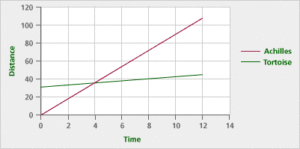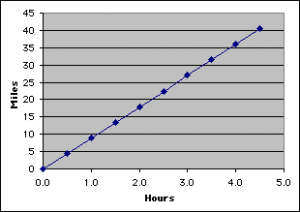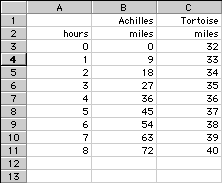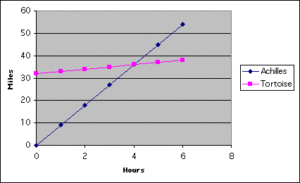# Linear Functions and Slope Part C: Rates (40 minutes)

## Session 5, Part C

A rate describes how much one variable changes with respect to another. Rates are often used to describe relationships between time and distance. When an object or person moves at a constant rate, the relationship between distance and time is linear. Note 11

Problem C1

Achilles runs at a constant rate of 9 miles per hour. Note 12

1. Write an equation describing the relationship between the distance Achilles covers and the time he runs.
2. How far will Achilles travel in 1.5 hours?
3. If you graphed the relationship between the distance Achilles covers and the time he runs, what would the graph look like?
4. Enter your equation in a spreadsheet, and use the spreadsheet to draw the graph. Is the graph what you expected? Explain why or why not.

Tip: Would a closed-form or a recursive rule be easier to work with in this situation?

Problem C2

Achilles is going to race against a tortoise, who moves at only 1 mile per hour. To make the race fair, the tortoise gets a head start of 32 miles.

1.  Write an equation describing the relationship between distance and time for the tortoise.
2. Enter the tortoise’s equation into a spreadsheet.
3. How long will it take for Achilles to catch up to the tortoise?

 Video Segment In this video segment, participants use a spreadsheet program to answer Problem C2. Watch this segment after you have completed Problem C2. If you get stuck on the problem, you can watch the video segment to help you. Could the participants have answered Problem C2 using a recursive rule? You can find this segment on the session video, approximately 17 minutes and 56 seconds after the Annenberg Media logo.

Problem C3

Make a single graph that shows the progress of Achilles and the tortoise. Where do the two lines cross?

Problem C4

What is the relationship between the points where the lines cross and Achilles passing the tortoise?

Problem C5

Which of the two lines in Problem C3 represents a proportional relationship? How do you know?

Tip: If you need more help with proportional relationships, refer to Session 4, Part C.

Problem C6

Suppose that two people were traveling a distance of 100 miles at the same speed, and the first person got a head start of 25 miles. When would you expect them to be at the same point? What does this tell you about their distance graphs? Note 13

### Notes

Note 11

The exercises in this part of the session link the concept of direct variation, covered in Session 4, to the idea of problem solving, which will be covered in Session 6.

Note 12

Groups: Work in pairs on Problems C1 and C2.

Consider sketching the situations or drawing tables to help come up with the equations for Achilles and the tortoise. After generating graphs for these situations, compare the graphs with the toothpick graph developed in Part A. In the case of Achilles and the tortoise, non-integer values make sense, so there is no problem with drawing connected lines between points.

The graph should look like this:Note 13

Problem C6 addresses the idea of parallel lines. You might want to refer to the graphs drawn in Part B. Ask if lines with the same slope ever intersect.

Students and teachers often miss the essential connection between solving linear equations and finding the intersection of lines. The intersection of two lines happens at an (x, y) pair that satisfies both linear equations. At this point in each equation, the xs are equal and the ys are equal. To find where this happens, set the ys equal to each other and solve each equation for x.

When trying to solve an equation like 5x = 5x + 25 by the usual method of “doing the same thing to both sides,” students end up with the equation 0 = 25 and don’t know how to interpret it. The point is that the two lines are parallel, so they never intersect, which means there is no solution to the equation. And the equation 0 = 25 is never true. Solving equations is covered more completely in the next session, but if there is time, think about some of these ideas now.

### Solutions

Problem C1

1. The equation A = 9h describes the distance in miles that Achilles travels, in terms of time measured in hours. “A” stands for the distance run by Achilles, while “h” stands for hours.
2. For this problem, h = 1 1/2, so A = 9(1 1/2) = 13 1/2 miles.
3. The graph should be a line through the origin, since the relationship is a proportional one.
4. The graph is a line through the origin. You should find that the line has a constant slope, or rate of change, of 9 miles per hour.Problem C2

1. The equation is T = h + 32. There is an invisible “1” in front of h, since the tortoise runs at 1 mile per hour.
2. As in Problem C1, you should find a constant rate; this time, the rate is 1 mile per hour.
3. A comparison of the spreadsheets finds that after exactly 4 hours, both Achilles and the tortoise are 36 miles from the start.Problem C3

According to the graphs, the intersection of the two lines occurs at the point (4, 36). Note that the independent variable (on the horizontal axis) represents time, and the dependent variable (on the vertical axis) represents distance.Problem C4

At the time h = 4, the distance for both Achilles and the tortoise is 36 miles. Since the graph represents both travelers’ positions over time, the two are at the same point at h = 4. It’s at this time that Achilles overtakes the tortoise.

Problem C5

Achilles’ graph is the proportional relationship because it is the graph of a line passing through (0, 0).

Problem C6

Because the two people are traveling at the same speed, the person with the 25 mile lead will keep that lead, at exactly 25 miles, for the entire race. The two distance graphs will never intersect, no matter how long the race is, which suggests that the graphs will be parallel. So, linear graphs with the same rate of change will be parallel.